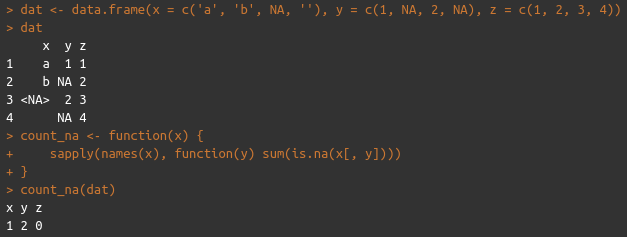## How to count the number of NA values in each variable of a data frame in R

19 January, 2022

This is one of those things that I find myself doing all the time, particularly after join operations of large datasets. Things can easily go wrong, and if you've used R for any length of time, you'll know that NAs have a habit of propagating—so it's always a good idea to keep a close eye on them.

This is essentially a one-line operation:

``sapply(names(x), function(y) sum(is.na(x[, y])))``

where `x` is the name of your data frame. This will return a named integer vector, where the element names are the names of the variables, and the integer values are the number of NAs in that particular variable vector.

It doesn't need to be a function, but I've wrapped it up in one and added it to my package of helpful little R snippets, to save my poor fingers a few seconds of typing. Like this:

``````count_na <- function(x) {
sapply(names(x), function(y) sum(is.na(x[, y])))
}``````

And here's an example of how it works:Feel free to install this package (the source code is available here) either by building from source, or with devtools: `devtools::install_gitlab("hedsnz/helprs")`. Once installed, you can access the function with `helprs::count_na(...)` (or by the typical `library` call).